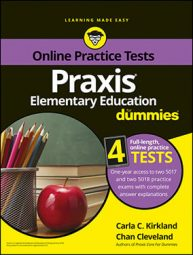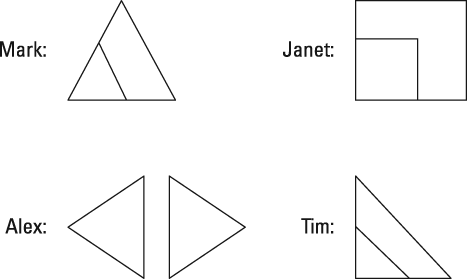##### Praxis Elementary Education For Dummies with Online Practice TestsThe Praxis Elementary Education exam will probably include a question that involves transformations—that is, changes in the positions or sizes of shapes. There are four major types of transformations, as you can see here.

A translation is the moving of a shape from one place to another. A reflection is the forming of a reverse image of a shape in reference to a line, which does not have to be shown. Dilation takes place when a shape gets bigger or smaller without changing shape. A rotation is the spinning of a shape around a point, as if the shape is on a bicycle spoke of a tire that is being spun.

## Practice question

1. A teacher assessed the work of four students she asked to use two figures each to demonstrate a certain geometric concept. Each of the following sets of figures represents the work of the student whose name corresponds to the set. Three of the students correctly demonstrated the geometric concept, and one student did not. The student with the incorrect answer demonstrated which of the following?A. reflection instead of dilation B. translation instead of reflection C. dilation instead of reflection D. translation instead of rotation

## Answer and explanation

1. The correct answer is Choice (A). Reflection involves a figure's transformation to a position in which it forms the reverse image of its original position in relation to a line. The line does not have to be illustrated. Dilation involves a figure's transformation to a similar figure of a different size. Alex demonstrated reflection, while the other three students demonstrated dilation.